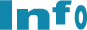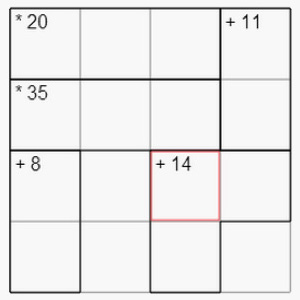﻿ Recreational and Educational Value of Math Puzzles
 EinloggenMitglied werden# Recreational and Educational Value of Math Puzzles

Tuesday, September 3, 2013Many people enjoy working on grid puzzles as small, quick challenges of their mathematical and logical skills. Here is one you may not have seen, the OkiDoku. How does it work? Looking at the grid above, try to find four different numbers and put them in these 16 squares in a way that will satisfy the following two conditions:

1. Each of these four numbers must appear exactly once in each row and in each column.

2. The blocks with thick borders are called cages. Each cage shows a target number and a mathematical operation. The operation applied to the numbers in the cage should produce the target number. For example, there is a cage in the first row with a target number of 20 and a mathematical operation of multiplication. The puzzle solver should put three numbers in the cage so that the product of these numbers is 20.

Give it a try.

This article was originally published on Edutopia.org of The George Lucas Educational Foundation. The figure above shows a puzzle called OkiDoku by Prof. Dani Novak & Prof. David Rosenthal, Ithaca College

The most famous of all grid puzzles is the Sudoku, a logic puzzle found in a vast majority of newspapers. Some of you may have encountered a mathematical grid puzzle called KENKEN® that appears in more than 100 national U.S. publications. KENKEN® was invented by Japanese teacher Tetsuya Miyamoto and introduced in the United States by NexToy, Inc.

Professors Dani Novak and David Rosenthal of Ithaca College have created a similar puzzle called OkiDoku and used it to make learning math an enjoyable experience. Several other grid puzzles appear at a popular puzzle website called ConceptisPuzzles. On the average, a whopping 20 million puzzles developed by this website are solved every day by adults and kids around the world. Clearly, there are many people who choose to solve grid puzzles as a recreational activity.

## Motivation to Learn

Because many students enjoy working on these puzzles, they can be easily motivated to adopt learning strategies that will improve their puzzle-solving skills. Grid number puzzles provide strong intrinsic motivation to solve for unknown numbers from a handful of clues. As many math problems have a similar form, students who enjoy solving these puzzles can develop positive attitudes toward other forms of math in non-puzzle contexts as well. I have taught puzzle math to students in grades three to six. In these classes, I have found that students show a significant positive change in their attitudes toward math after a year of recreational math activities. Professor Harold Reiter of University of North Carolina, along with Professor Novak of Ithaca, also found that teaching puzzle math has a positive influence on student attitudes towards math.

## Educational Value of Puzzles

Even without supervision, students can learn to be creative and persistent after working on many hard grid puzzles. In my classes, I augment self-exploration of grid puzzles with a guided exploration that teaches problem-solving, reflective learning and algebra techniques.

Let me illustrate this with the puzzle shown at the beginning of this post. Even though we can use the creative problem-solving and logical reasoning approaches that we usually use in solving puzzles, we can progress a bit faster on the above puzzle with some help from algebra.

Suppose the top number in the 11+ cage is x and the bottom number in the same cage is y.

We know that each row has to have the same four numbers. So the product of all numbers in each row is the same. As the product of first three numbers in the first row is given to be 20 and the fourth number is x, the product of all numbers in the first row is 20x. As the product of the first three numbers in the second row is given to be 35 and the fourth number is y, the product of all numbers in the second row is 35y. As the product of all numbers in the first row is the same as the product of all numbers in the second row, we know that 20x = 35y. We have also been given the clue in the 11+ cage that x + y = 11.

So what we have is a set of equations:

• 20x = 35y
• x + y = 11

This is now an algebra problem. The use of algebra to solve a problem that students are deeply engaged in allows them to appreciate the power of algebra and also provides a strong motivation to study algebra.

I have taught a yearlong extracurricular class that covers a variety of math problem-solving techniques and algebra in the context of grid puzzles. These techniques include:

• Making a table
• Divisibility rules
• Multiplication tricks
• Sets
• Venn Diagrams
• Factors
• Logical charts
• Logical reasoning
• Working backward
• Arithmetic sequences
• Case-based reasoning
• Algebra

I have written three books that can be used to learn problem solving with grid puzzles. Additional techniques on grid puzzles are described at the Math Olympiad site.

## Effectiveness of Puzzle Math in Improving Student Performance

In my classes, I teach math problem solving in the context of puzzles, letting students practice their skills with puzzles and finally encouraging them to ensure their mastery by applying their skills in other math word problems. I have found that students in my class show remarkable improvement in their math problem-solving and learning abilities. However, skill improvement among students varies significantly depending on other factors such as the amount of effort students put into doing homework, as well as student aptitude. In a national Noetic math contest, a majority of students in my puzzle math class showed significant improvement in math skills. Two students were perfect scorers, and a significant percentage of students won national recognition.

While puzzle math is valuable for achieving certain educational objectives, it may not be the best choice in all educational situations. For example, if students and teachers have short-term objectives to achieve the best results on particular tests with specific types of questions, then practicing questions similar to those on the tests can help them achieve those objectives -- not the approach described above. Similarly, teachers may want to emphasize the relationships of math concepts to real-world applications. Again, puzzle math would not support this objective.

In short, teachers will find the puzzle math approach useful to help achieve certain objectives but not others. However, there is no question that teaching math problem solving in the context of grid puzzles, long regarded as a recreational activity, is effective in cultivating students' interest in math and in improving their problem-solving, reflective learning and algebra skills.